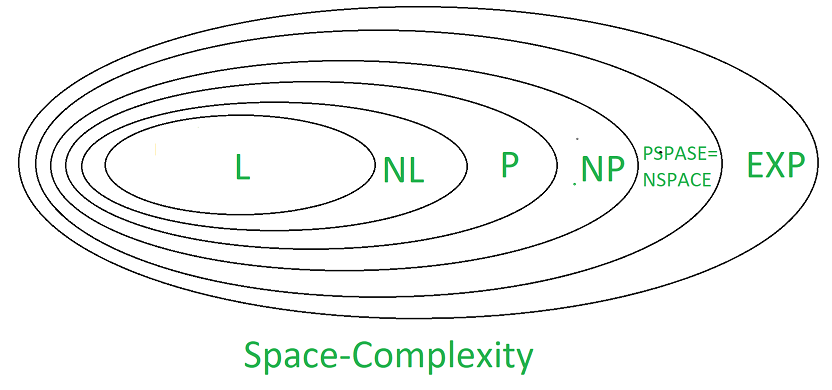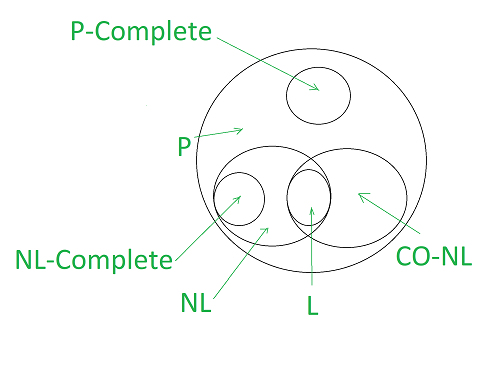# NL-Completeness and PSPACE-Completeness

• Last Updated : 05 Nov, 2021

For solving complex problems we require efficient algorithm. We face so many problems and some of them can be solved by using an algorithm and every algorithm requires some space and time. For better performance of program we need to reduce space and time complexity of algorithm.

Examples which will take more memory: the web graph, genomes etc.

We want algorithm which uses small amount of memory, so that large amount of data can be manipulated without storing all data to computer hard-disk at a time.  An algorithm take sub linear space because input n bits take take linear space.

Two-tape Turing machine is used here-

1. Read- only tape containing input
2. Work-tape that can be freely used

Space acquired by work tape contribute to space complexity.

We have,

``` L = SPACE ( log n ) , and
NL = NSPACE ( log n ) ```

SPACE COMPLEXITY :
It is defined as the amount of computer memory space required to solve any given computational problem as function of input or we can say that it is the amount of  memory needed by an algorithm ,until its execution complete.  An algorithm is called complete if it will terminates and if its solution exist.NL-Completeness :
NL contains decision problem and solved by Non Deterministic Turing Machine. Before understanding NL-completeness first we understand Log space-Reducibility.

Log space-Reducibility :
A is log space reducible to B, if for all function f :

` {0, 1}* → {0, 1}* such that x ∈ A if f (x) ∈ B for every x ∈ {0, 1}* `

For defining NL completeness , we requires suitable reduction. Whether L = NL is the complexity theoretic question. Hence reduction is log space reduction, i.e. given an input string x , we have a function f(x) such that f(x) is computable by DTM by using at most O(log |x|) cells in the work tape.

A language “A” is NL-complete  if it satisfy the two conditions below-

1. “A” is in NL. (A ∈ NL)
2. Language belong to NL  can be log space reducible to “A”

(for all B in NL, there exist B ≤L  A).

Applications :

• ST-connectivity
• Propositional Logic
• 2-satisfiability

PSPACE- completeness :
All the decision problems  that can be solved in polynomial input length, and if every other problem solved in polynomial space can be converted to polynomial time. The “word problem” for deterministic context-sensitive grammars was the first PSPACE complete problem.

A language “A” is PSPACE-complete  if it satisfy the two conditions below

1. “A” is in PSPACE. (A ∈ PSPACE)
2. Language belong to PSPACE can be polynomial time reducible to “A”

(for all B in PSPACE, there exist  B ≤L  A).

Note that if “A” is following only second condition then we say it is PSPACE-hard.

Applications :

• Hex(board game).
• First order logic of equality
• First order theory of well-ordered sets
• Lambda calculus etc.Why log space reduction is used for NL-completeness while PSPACE reduction is not used for PSPACE-completeness?
As  L ⊆  NL  ⊆ P . therefore it doesn’t make sense to use polynomial time reduction for NL-completeness, since poly time reduction is more powerful than the class L. hence we use log space reduction for NL-completeness.

Completeness results are  more impressive using weaker reduction since reduction is able to do less of the work ,hence  reduction is actually doing all work hence we use polynomial time reduction instead of polynomial space-reduction.

My Personal Notes arrow_drop_up
Recommended Articles
Page :